## On his way to deliver presents Santa has a minor accident. If the sleigh (1200 kg) was traveling at 322 m/s and the jet(4800 kg) was traveli

Question

On his way to deliver presents Santa has a minor accident. If the sleigh (1200 kg) was traveling at 322 m/s and the jet(4800 kg) was traveling at 680 m/s and they collided head on, what was the final velocity of the two objects after the collision

in progress 0
2 weeks 2021-08-29T00:06:07+00:00 1 Answers 0 views 0

608.4m/s

Explanation:

We are given that

Mass of Sleigh,M=1200 kg

Speed of Sleigh,u=322 m/s

Speed of jet,u’=680 m/s

Mass of jet,m=4800 kg

Total mass=M+m=1200+4800=6000 kg

We have to find the final velocity of the two objects after the collision.

The collision is inelastic .

By using law of conservation of momentum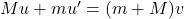Using the formula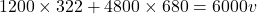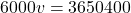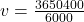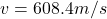Hence, the final  velocity of two objects after the collision=608.4m/s